# Study Guide

## Field 019: Physics Sample Multiple-Choice Questions

The following reference material will be available to you during the test:

Constants#### Subarea I1—FOUNDATIONS OF SCIENTIFIC INQUIRY

##### Objective 001Understand the principles and procedures of scientific inquiry.

1. Use the diagram below to answer the question that follows.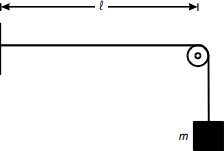The diagram shows a horizontal string, connected at one end to a stationary vertical surface and at the other end to a weight of mass m hung over a cylinder. The distance from the vertical surface to the center of the cylinder is dimensioned as l.

The diagram shows a string from which different masses may be suspended. The pitch of the note produced when the string is plucked is measured using an electronic tuning device. Which of the following is the dependent variable in this experiment?

1. frequency
2. mass
3. wavelength
4. amplitude
Correct Response: A.

##### Objective 002Apply knowledge of methods and equipment used in scientific investigations.

2. Use the diagram below to answer the question that follows.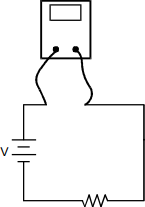The meter in the circuit above is positioned to measure:

1. charge stored in the resister.
2. current through the resister.
3. impedance of the resister.
4. potential difference across the resister.
Correct Response: B.

##### Objective 003Understand the development of scientific thought and inquiry.

3. In the 1930s Lise Meitner proposed a mathematical theory that explained how barium was produced when a uranium nucleus was struck by a neutron. Which of the following best describes the significance of this work?

1. It described the process that would later be used to develop atomic energy.
2. It provided the basis for Einstein to develop the theory of relativity.
3. It described the processes of mass-energy transformations that occur in stars.
4. It verified earlier theories proposed by Fermi and Oppenheimer.
Correct Response: A.

#### Subarea II2—MECHANICS

##### Objective 006Analyze motion in one and two dimensions.

4. A wheel of radius 0.5 m rotates with an angular speed of 4 rad/s. What is the linear speed of a point on the outer rim of the wheel?

1. 2 m/s
2. 4 m/s
3. 8 m/s
4. 16 m/s
Correct Response: A.

Use the diagram below to answer the two questions that follow.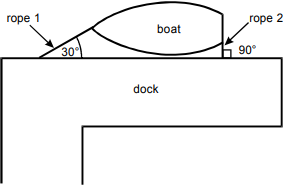The diagram shows a boat tied parallel to a dock. Rope 1 ties the bow to the dock, meeting the dock at a 30 degree angle. Rope 2 ties the stern to the dock, meeting the dock at a 90 degree angle.

The diagram shows a small boat at a dock in a river with a current flowing. The boat is tied to the dock by rope 1 at the front end and by rope 2 at the back end. The tension in rope 1 is 200 N at an angle of 30 degrees with the dock. The tension in rope 2 is 73 N perpendicular to the dock.

##### Objective 007Understand Newton's laws of motion and the law of universal gravitation.

5. What is the magnitude of the force from the current on the boat?

1. 127 N
2. 173 N
3. 245 N
4. 283 N
Correct Response: C.

6. Which of the following could represent the direction of the force from the current on the boat?

1.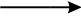2.3.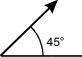4.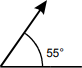Correct Response: C.

##### Objective 008Understand conservation of energy and conservation of momentum.

7. Use the diagram below to answer the question that follows.The diagram shows before and after states of two disks on an X, Y graph. In the before state, disk P is to the left of disk Q, moving horizontally to the right at a velocity marked as 2.0 m/s. In the after state, disk P is in a position to the right and below the original position of disk Q, and disk Q is moving at a 30° angle at a velocity marked as 1.7 m/s.

Two identical disks on a frictionless surface collide. Initially, disk Q is at rest and disk P has a horizontal velocity of 2.0 m/s. After the collision, disk Q has a velocity of 1.7 m/s in the direction shown. What is the y-component of the velocity of disk P after the collision?

1. negative 0.85 m/s
2. negative 1.0 m/s
3. negative 1.5 m/s
4. negative 1.7 m/s
Correct Response: A.

##### Objective 009Understand torque, static equilibrium, and rotational dynamics.

8. Use the diagram below to answer the question that follows.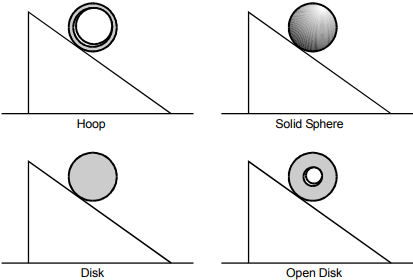The diagram shows 4 inclines. Each incline has an object rolling down the slope. Each object is at the same position on its incline. The objects are labeled hoop, solid sphere, disk, and open disk.

A hoop, a solid sphere, a disk, and an open disk all have the same mass and radius and roll without slipping down an incline. Which one will have the least acceleration?

1. the hoop
2. the solid sphere
3. the disk
4. the open disk
Correct Response: A.

##### Objective 010Understand the characteristics of oscillatory motion.

9. Use the graph below to answer the question that follows.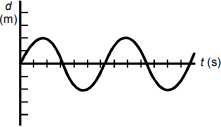The vertical axis is labeled d, in meters, and the horizontal axis is labeled t, in seconds. The data plot is a sinusoidal curve beginning upward from the origin. The curve reaches vertical values of 2 units and negative 2 units and crosses the t axis every 3 units.

The graph shows the displacement, d, of a mass as a function of time. Which of the following graphs shows the velocity, v, of the mass as a function of time?

1.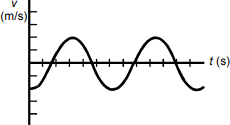The vertical axis is labeled v, in meters per second, and the horizontal axis is labeled t, in seconds. The same data plot is shown, starting at negative 2 on the vertical axis.

2.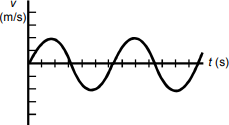The vertical axis is labeled v, in meters per second, and the horizontal axis is labeled t, in seconds. The same data plot is shown, starting upward from the origin.

3.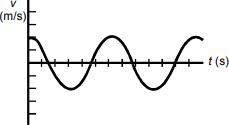The vertical axis is labeled v, in meters per second, and the horizontal axis is labeled t, in seconds. The same data plot is shown, starting at 2 on the vertical axis.

4.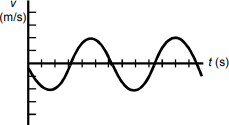The vertical axis is labeled v, in meters per second, and the horizontal axis is labeled t, in seconds. The same data plot is shown, starting downward from the origin.
Correct Response: C.

#### Subarea III3—ELECTRICITY AND MAGNETISM

##### Objective 011Understand electric charge, electric fields, and electric potential.

10. Which of the following diagrams correctly represents the lines of equipotential for the electric field between the two charged parallel plates of a capacitor?

1.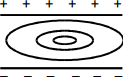The diagram shows two horizontal plates in cross-section. The upper plate is marked with plus signs, and the lower plate is marked with negative signs. The field lines are concentric horizontal ellipses.

2.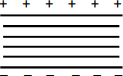The diagram shows two horizontal plates in cross-section. The upper plate is marked with plus signs, and the lower plate is marked with negative signs. The field lines are parallel horizontal lines.

3.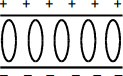The diagram shows two horizontal plates in cross-section. The upper plate is marked with plus signs, and the lower plate is marked with negative signs. The field lines are a series of vertical ellipses of equal size.

4.The diagram shows two horizontal plates in cross-section. The upper plate is marked with plus signs, and the lower plate is marked with negative signs. The field lines are parallel vertical lines.
Correct Response: B.

##### Objective 012Understand simple circuits.

11. A flashlight consists of two 1.5 V batteries and a lamp wired in series. The illuminated lamp has a resistance of 20  ohms. How much charge passes through the lamp each minute?

1. 0.15 C
2. 6.7 C
3. 9.0 C
4. 400 C
Correct Response: C.

##### Objective 013Understand magnetic fields.

12. The magnetic field inside a solenoid of length L with 100 turns of wire carrying a current I has magnitude B. If the number of turns of wire is doubled while L and I remain constant, the magnitude of the field will be:

1.B.
2.B.
3. 2B.
4. 4B.
Correct Response: C.

##### Objective 014Understand electromagnetic induction.

13. Use the diagram below to answer the question that follows.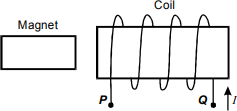The diagram shows a bar magnet positioned to move through the center of a coil of wire. The bottom right point of the coil, hanging down from behind the magnet, is labeled Q. To the right of point Q there is an arrow pointing up, labeled I. The bottom left point of the coil, hanging down from in front of the magnet, is labeled P.

The diagram above shows a bar magnet to the left of a coil of wire. What is the orientation and the direction of motion of the magnet with respect to the coil to induce a current that flows from point Q to point P at the time shown?

1.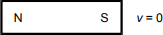2.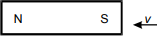3.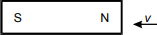4.Correct Response: C.

#### Subarea IV4—WAVES, ACOUSTICS, AND OPTICS

##### Objective 015Understand the characteristics of waves and wave motion.

14. Use the graph below to answer the question that follows.The vertical axis is labeled Displacement X, in meters, with values marked from negative 2.0 to 2.0 in increments of 1.0. The horizontal axis is labeled time t, in seconds, with values marked from 0 to 5.0 in increments of 1.0. The data curve is a sinusoidal wave beginning upward from the origin, reaching displacement values from 1 meter to negative 1 meter, and crossing the horizontal axis every 1.0 seconds.

Waves of velocity 4.0 m/s travel along a rope. The graph shows the displacement of a single point on the rope as a function of time. Which of the following is the wavelength of the waves on the rope?

1. 1.0 m
2. 2.0 m
3. 4.0 m
4. 8.0 m
Correct Response: D.

##### Objective 016Understand the principles of sound and acoustics.

15. A stationary source is producing sound with a frequency ƒ = 500 Hz. The velocity of sound in air is 343 m/s. An observer approaches the source at 20 m/s. What pitch is heard by the observer?

1. 235 Hz
2. 472 Hz
3. 529 Hz
4. 728 Hz
Correct Response: C.

##### Objective 018Understand ray optics.

16. Use the mirror equation below to answer the question that follows.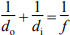An object is located 5.0 cm in front of a concave mirror whose focal length is 20.0 cm. Where is the image located?

1. 4.0 cm in front of the mirror
2. 5.7 cm behind the mirror
3. 6.7 cm behind the mirror
4. 8.0 cm in front of the mirror
Correct Response: C.

#### Subarea V5—NATURE OF MATTER, THERMODYNAMICS, AND MODERN PHYSICS

##### Objective 019Understand the particulate nature of matter.

17. Which of the following is a feature of the Maxwell-Boltzmann theory of an ideal gas?

1. The speeds of the gas molecules are distributed about an average determined by temperature.
2. The overlapping wavelengths of the gas molecules result in a predictable relationship between pressure and volume.
3. The energy levels of the gas molecules are only allowed to be in discrete, quantized states.
4. The gas molecules are modeled as an ensemble of weakly interacting quantum mechanical harmonic oscillators.
Correct Response: A.

##### Objective 020Understand the laws of thermodynamics.

18. A resistor submerged in 200 g of water at 20 degrees C is connected to a 10 V DC power source. A current of 0.5 A is applied to the resistor. Assuming the system is thermally insulated, which of the following expressions represents the temperature increase of the water after 5 minutes (specific heat of water = 4.18 J/g degrees C)?

1.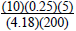2.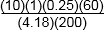3.4.Correct Response: D.

##### Objective 021Understand the basic ideas of quantum mechanics and relativity.

19. Photons of energy 4.98 e upper V are incident on a metal with a work function of 4.73 e upper V. What is the maximum kinetic energy of an electron emitted from the metal?

1. 0.25 e upper V
2. 1.05 e upper V
3. 4.85 e upper V
4. 9.71 e upper V
Correct Response: A.

##### Objective 022Understand the basic ideas of nuclear physics.

20. Which of the following best describes the principle of magnetic resonance imaging (MRI) used in medical research?

1. mapping the magnetic field generated by electron transitions in molecules
2. measuring the energy absorbed as nuclear magnetic moments flip between spin states
3. using a magnetic field to focus the paths of emissions from a radioisotope
4. producing high-energy electromagnetic waves using superconducting magnets